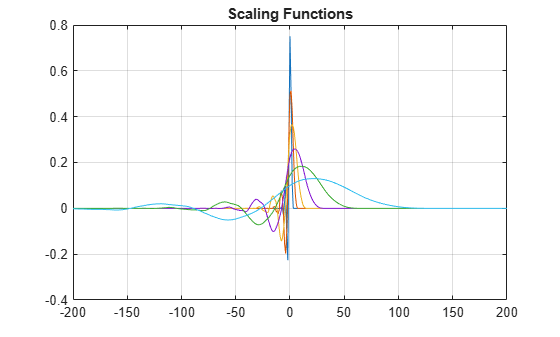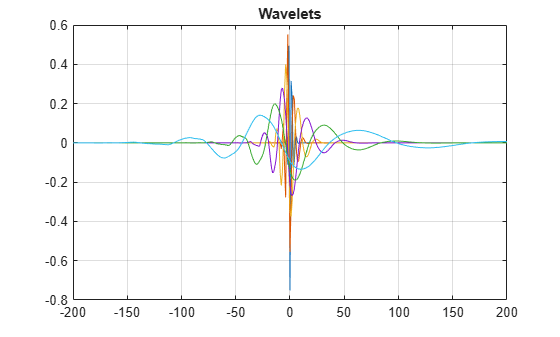Main Content

# isOrthogonal

Determine if DWT filter bank is orthogonal

## Syntax

``tf = isOrthogonal(fb)``
``tf = isOrthogonal(fb,tol)``

## Description

example

````tf = isOrthogonal(fb)` returns `true` if the discrete wavelet transform (DWT) filter bank `fb` is an orthogonal filter bank and `false` otherwise.To determine if a DWT filter bank is biorthogonal, use `isBiorthogonal`.```
````tf = isOrthogonal(fb,tol)` uses the positive real-valued tolerance `tol` to determine the orthogonality of the filter bank `fb`. `tol` is a small positive number in the interval (0,10-2]. If unspecified, `tol` defaults to 10-5.```

## Examples

collapse all

Create a DWT filter bank using the Daubechies `db6` wavelet. Confirm the filter bank is orthogonal.

```fb = dwtfilterbank('Wavelet','db6'); isOrthogonal(fb)```
```ans = logical 1 ```

Plot the time-domain and centered scaling functions for each level in the filter bank.

```[phi,t] = scalingfunctions(fb); psi = wavelets(fb); plot(t,phi') grid on xlim([-200 200]) title('Scaling Functions')```Confirm the scaling functions have norm square equal to 1.

`sum(phi.^2,2)`
```ans = 6×1 1.0000 1.0000 1.0000 1.0000 1.0000 1.0000 ```

Plot the time-domain and centered wavelets corresponding to the wavelet passband filters.

```plot(t,psi') grid on xlim([-200 200]) title('Wavelets')```Confirm the wavelets have norm square equal to 1.

`sum(psi.^2,2)`
```ans = 6×1 1.0000 1.0000 1.0000 1.0000 1.0000 1.0000 ```

## Input Arguments

collapse all

Discrete wavelet transform (DWT) filter bank, specified as a `dwtfilterbank` object.

Tolerance to use to determine orthogonality of the filter bank, specified as a positive scalar in the interval (0,10-2].

## See Also

Introduced in R2018a

## SupportGet trial now